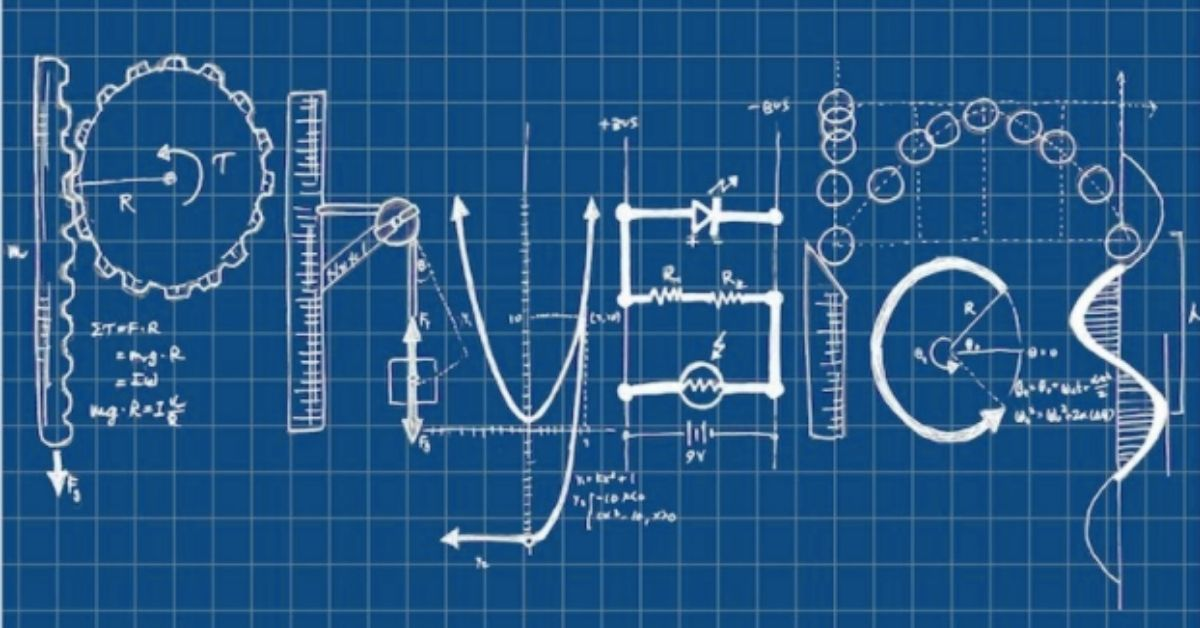# Becoming Much Smarter in PhysicsSteve Schecter
February 23, 2015

For most students, the key to the game of science is to overcome feelings of intimidation. Many students believe and feel that science is difficult, and so they never learn how to approach the game and make it their own. Instead they muddle through. The most intimidating of the sciences for high school students appears to be physics, so I’d like to offer a few thoughts on how to get started with physics and take the first steps toward mastery.

Learning the game of physics is similar to learning the game of math. In fact, physics is math – applied to describing and understanding the physical world. The game consists of a number of equations that describe relationships in the world. These equations help us understand how things move, how they rise and fall, what happens when you push and pull things. These equations also help us understand phenomena such as sound, light, electricity, magnetism, and nuclear energy.

Physics is all about creating and using models. The key to creating a model is to capture the essentials of the thing being studied, and to ignore some details. For example, Galileo, centuries ago, posed the idea that a light object, such as a feather, and a heavy object, such as a rock, would fall from a height in much the same way. This idea did not make sense to Galileo’s contemporaries, because it seemed to them that a rock, if dropped from a height, would fall to the ground much faster than a feather. Galileo created a model by observing the essential relationship between the way a rock and a feather would fall: they would both be pulled to earth by gravity. He also created a model by ignoring what was different about the ways a rock and feather would fall: that air resistance would slow the descent of the feather much more than it would slow the descent of the rock.

So, physics is a series of models, defined by equations, that describe the way much of our physical world works.

The keys to learning physics are to understand the models, and to learn how to solve physics problems.

To understand the models, be willing to play badly at first. Some of the models are hard to understand when they are introduced to you verbally. So what you do is observe a model, understand as much of it as you can, and then look at some sample physics problems, with demonstrated solutions, related to that model. Try these problems for yourself. You’ll find that, doing the problems yourself, even with the help of demonstrated solutions, will make you start to understand the model. Then go back and re-read the model; then do some more problems. Soon, you’ll “get” the model.

Another aspect of excelling in high school physics is solving physics problems. If you are taking an introductory high school physics course, you will most likely be able to rely on algebra and not need calculus. Algebra provides the core skill set for solving physics problems. Algebra is the art of using what you know to find out what you don’t know. To solve a physics problem, you note down what you know, and what you are asked to find out. You make a sketch so that you can “see” the physical problem, what you know, and what you don’t know. Then you ask yourself, “Which formula or formulas can I use?” It’s not all that hard to choose the right formula: it will use the information that you have been given!

As you would learn any other game, start learning physics by taking on simple problems. Get started with the process of taking down what you know, making a sketch, and finding a formula that will solve your problem. You will soon find that you can take on more complicated problems that require several steps and use several formulas.

So the key to getting started with physics is to use the Much Smarter method. To build the learning muscle in physics:

1. Approach the learning as a game
2. Be willing to play badly at first
3. Get the whole story up front (how does this game work and how does one play well?)
4. Learn the moves of the game (in this case, the models and the different types of physics problems)
5. Practice the moves
6. Refine the moves
7. Practice again

Approaching physics (and other branches of science) in this way will enable you to get past intimidation and move toward mastery!

- Steve